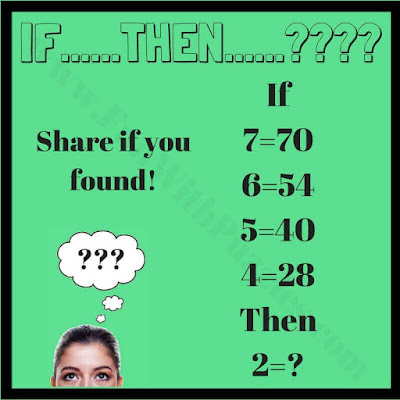## Friday, September 25, 2020

Mathematics Equations are very much fun to solve. However, if we twist these mathematical equations then the fun of solving it with logic gets double. Here are some math equations which has been twisted so that the result is different from the expected answer. However, each equation in every brain teaser below follows some logical twist. One has to find this logical twist to these math equations and has to calculate the final equation so that they follow the same logic as the previous equations.
Answers to these equations along with explanations are given below. However please do post your answers with logical conclusions in the comment box of this post.1. Maths Logical Equation Puzzle2. Logical Math Puzzle3. Logical Thinking Math Equations4.  Math Brain Teaser5. Math IQ Question

These Mathematics Logic Brain Cracking Problems are part 1. Next set of similar logical Maths problem can be found at Mind Challenging Maths Logical Questions and Answers.

Do check more Mathematical and Logical puzzles as listed below
List of Mathematical & Logical Brain Teasers
1. Mind Twisting Out of Box Thinking Brain Teasers with Answers and Explanations: It contains 5 Mind Twisting Brain Teasers which will make you think out of the box.

2. Interesting Brain Teasers to Challenge your Mind: It contains very interesting mathematical, logical and picture brain teasers to challenge your mind.

3. Easy Mathematical Brain Teasers with answers: Here you can find easy Mathematical Brain Teasers for kids and teens.

4. Math Logic Brainteasers for high school students: If you liked above given Mathematics Logic Brain Cracking Problem, then you should not be missing these Math Logic Brainteasers.

1.1. (4+20)×3=72
2. 2*6=12
3. (7*3)(7+3)=2110
4.8*(11+1)=96
5. 72
6. (9+5)*5=70
7. (1*3)+1+3=7
8. 7
9. 6+3 (as 7 square is 63) sum of digits of the square of number
10. (10*9)+9-1=98 or
(10+1)*9-1=98

1.I tought number 2 was 2*5=10

2.And also square of 7 is 49 so its 4+9 = 13

3.Thanks Sumitra,
Most of your answers are correct. Now we have updated answers of these Mathematical Logic Brain Cracking Problem along with the explanation.

2.number 3 is 2110

1.3.x^2+x
-----
x^2-1

4.45+54=92
56+65=14
34+43=91
22+33=?

A comment doesn't cost a thing. Please drop a comment below to boost the author's morale.